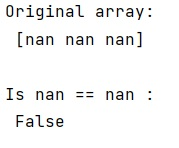# Why in NumPy 'nan == nan' is False while nan in [nan] is True?

Learn why in NumPy 'nan == nan' is False while nan in [nan] is True in Python?
Submitted by Pranit Sharma, on February 11, 2023

NumPy is an abbreviated form of Numerical Python. It is used for different types of scientific operations in python. Numpy is a vast library in python which is used for almost every kind of scientific or mathematical operation. It is itself an array which is a collection of various methods and functions for processing the arrays.

## Explanation – Why 'nan == nan' is False while nan in [nan] is True?

When it comes is nan==nan, it returns False because of a simple reason, nan is Not a Number, and is not equal to anything, including Not a Number.

As for nan in [nan] being True, that's because identity is tested before equality for containment in lists. We are comparing the same two objects.

Let us understand with the help of an example,

## Python code to demonstrate why 'nan == nan' is False while nan in [nan] is True

```# Import numpy
import numpy as np

# Creating a numpy array
arr =  np.array([np.nan,np.nan,np.nan])

# Display original array
print("Original array:\n",arr,"\n")

# Checking nan with ==
print("Is",arr,"==",np.nan,":\n",arr==np.nan,"\n")
```

Output: